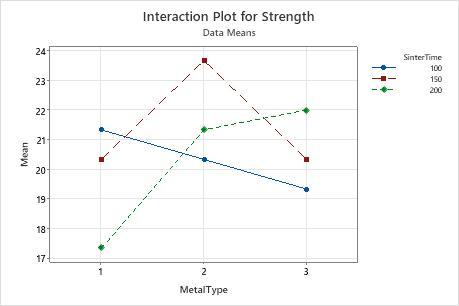# Example of Interaction Plot

An engineer wants to assess the effect of sintering time on the compressive strength of three different metals. The engineer measures the compressive strength of five specimens of each metal type at each sintering time: 100 minutes, 150 minutes, and 200 minutes.

The engineer performs a general linear model (GLM) ANOVA, and includes an interaction plot in the output.

1. Open the sample data, SinteringTime.MTW.
2. Choose Stat > ANOVA > Interaction Plot.
3. In Responses, enter Strength.
4. In Factors, enter SinterTime and MetalType.
5. Click OK.

## Interpret the results

The interaction plot shows the mean strength versus sintering time for each of the three metal types. The nonparallel lines on the interaction plot indicate interaction effects between metal type and sintering time. This interaction effect indicates that the relationship between metal type and strength depends on the value of sinter time.

If the engineer uses MetalType 2, SinterTime 150 is associated with the highest mean strength. However, if the engineer uses MetalType 1, SinterTime 100 is associated with the highest mean strength.

Although you can use this plot to display the effects, be sure to evaluate statistical significance by looking at the effects in an analysis of variance table.

This plot displays data means. While you can use the data means to obtain a general idea of which effects may be evident, it is generally good practice to use the fitted means in Factorial Plots to obtain more accurate results.By using this site you agree to the use of cookies for analytics and personalized content.  Read our policy